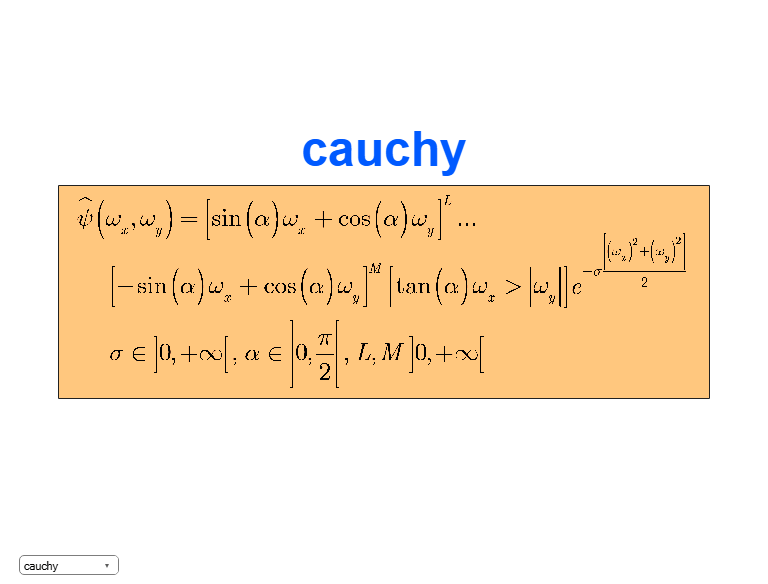# cwtftinfo2

Supported 2-D CWT wavelets and Fourier transforms

## Syntax

``cwtftinfo2``
``cwtftinfo2(wname)``

## Description

example

````cwtftinfo2` lists the supported 2-D continuous wavelet transform (CWT) wavelets and corresponding parameters for use with `cwtft2`.```

example

````cwtftinfo2(wname)` displays the equation for the 2-D Fourier transform of the wavelet, `wname`. The figure with the 2-D Fourier transform of the analyzing wavelet has a drop-down list from which you can select other wavelets.```

## Examples

collapse all

`cwtftinfo2`
``` CWTFTINFO2 Information on wavelets for CWTFT2 CWTFTINFO2 provides information on the available wavelets for 2-D Continuous Wavelet Transform using FFT. The wavelets are defined by their Fourier transform. The formulae giving the Fourier transform of the wavelet which short name (see below) is SNAME will be displayed using CWTFTINFO2(SNAME). The table below gives the short name of each wavelet and the associated parameters: first, the name of parameter and then the default value. WAV_Param_Table = {... 'morl' , defaults: omega0 = 6; sigma = 1; epsilon = 1; 'mexh' , defaults: p = 2; sigmax = 1; sigmay = 1; 'paul' , defaults: p = 4; 'dog' , defaults: alpha = 2; 'cauchy' , defaults: alpha = pi/6; sigma = 1; L = 4; M = 4; 'escauchy' , defaults: alpha = pi/6; sigma = 1; L = 4; M = 4; 'gaus' , defaults: p = 1; sigmax = 1; sigmay = 1; 'wheel' , defaults: sigma = 2; 'fan' , defaults: omega0 = 5.336; sigma = 1; epsilon = 1; J = 6.5; 'pethat' , defaults: No parameters. 'dogpow' , defaults: alpha = 1.25; p = 2; 'esmorl' , defaults: omega0 = 6; sigma = 1; epsilon = 1; 'esmexh' defaults: sigma = 1; epsilon = 0.5; 'gaus2' , defaults: p = 1; sigmax = 1; sigmay = 1; 'gaus3' , defaults: A = 1; B = 1; p = 1; sigmax = 1; sigmay = 1; 'isodog' , defaults: alpha = 1.25; 'dog2' , defaults = alpha = 1.25; 'isomorl' , defaults: omega0 = 6; sigma = 1; 'rmorl' , defaults: omega0 = 6; sigma = 1; epsilon = 1; 'endstop1' , defaults: omega0 = 6; 'endstop2' , defaults: omega0 = 6; sigma = 1; 'gabmexh' , defaults: omega0 = 5.336; epsilon = 1; 'sinc' , defaults: Ax = 1; Ay = 1; p = 1; omega0X= 0; Omega0Y = 0; }; The various wavelets may be grouped in families as follow: MORLET: 'morl' , 'esmorl' , 'rmorl' , 'isomorl' DOG: 'dog' , 'isodog' , 'dog2' , 'dogpow' GAUSS: 'mexh' , 'gaus' , 'gaus2' , 'gaus3' , 'esmexh' PAUL: 'paul' CAUCHY: 'cauchy' , 'escauchy' WHEEL: 'wheel', 'pethat' MISCELLANEOUS : 'endstop1' , 'endstop2' , 'gabmexh' , 'sinc' , 'fan' REFERENCES Two-Dimensional Wavelets and their Relatives J.-P. Antoine, R. Murenzi, P. Vandergheynst andS. Twareque Ali Cambridge University Press - 2004 Two-dimensional wavelet transform profilometry Fringe Pattern Analysis Using Wavelet Liverpool John Moores University http://www.ljmu.ac.uk See also CWTFT2 ```

Display the expression for the 2-D Fourier transform of the Cauchy wavelet. After displaying the Fourier transform for any wavelet, you can use the drop-down list in the bottom left to view the Fourier transform for any supported wavelet.

`cwtftinfo2('cauchy')`## Input Arguments

collapse all

Wavelet name, specified as a character vector or string scalar. The following table lists the supported wavelets for the 2-D CWT and associated parameters:

Wavelet nameParameters
`'morl'``{'Omega0',6;'Sigma',1;'Epsilon',1}`
`'mexh'``{'p',2;'sigmax',1;'sigmay',1}`
`'paul' ``{'p',4}`
`'dog'``{'alpha',1.25}`
`'cauchy'``{'alpha','pi/6';'sigma',1;'L',4;'M',4}`
`'escauchy'``{'alpha','pi/6';'sigma',1;'L',4;'M',4}`
`'gaus'``{'p',1;'sigmax',1;'sigmay',1}`
`'wheel'``{'sigma',2}`
`'fan'``{'Omega0X',5.336;'Sigma',1;'Epsilon',1;'J',6.5}`
`'pethat'`None
`'dogpow'``{'alpha',1.25;'p',2}`
`'esmorl'``{'Omega0',6;'Sigma',1;'Epsilon',1}`
`'esmexh'``{'Sigma',1;'Epsilon',0.5}`
`'gaus2'``{'p',1;'sigmax',1;'sigmay',1}`
`'gaus3'``{'A',1;'B',1;'p',1;'sigmax',1;'sigmay',1}`
`'isodog'``{'alpha',1.25}`
`'dog2'``{'alpha',1.25}`
`'isomorl'``{'Omega0',6;'Sigma',1}`
`'rmorl'``{'Omega0',6;'Sigma',1;'Epsilon',1}`
`'endstop1'``{'Omega0',6}`
`'endstop2'``{'Omega0',6;'Sigma',1}`
`'gabmexh'``{'Omega0',5.336;'Epsilon',1}`
`'sinc'``{'Ax',1;'Ay',1;'p',1;'Omega0X',0;'Omega0Y',0}`

Example: `cwtftinfo2('paul')`

Data Types: `char`

Introduced in R2013b

## SupportGet trial now